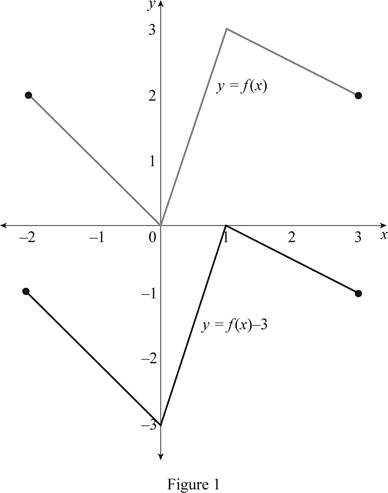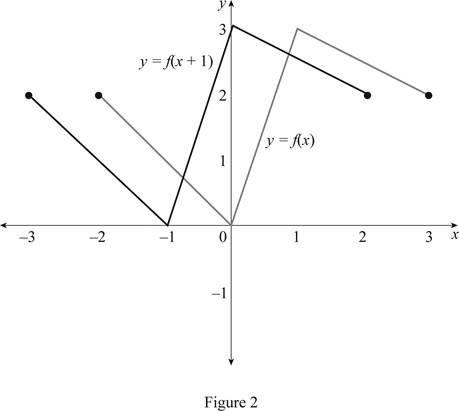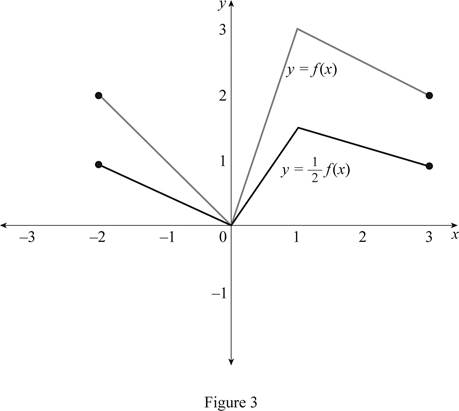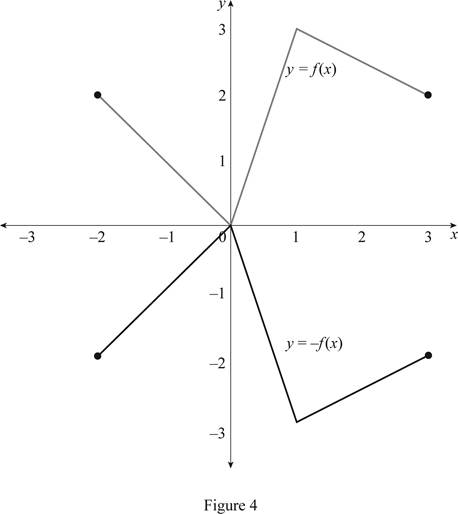# The graph of the function y = f ( x ) − 3 by using the given graph.### Single Variable Calculus: Concepts...

4th Edition
James Stewart
Publisher: Cengage Learning
ISBN: 9781337687805### Single Variable Calculus: Concepts...

4th Edition
James Stewart
Publisher: Cengage Learning
ISBN: 9781337687805

#### Solutions

Chapter 1.3, Problem 4E

(a)

To determine

## To sketch: The graph of the function y=f(x)−3 by using the given graph.

Expert Solution

### Explanation of Solution

The equation y=f(x)3 represents that the graph of the function is shifted 3 units downward. Thus, the graph of y=f(x4) is shown below in Figure 1.From Figure 1, it is observed that the actual curve is shifted 3 units downward.

(b)

To determine

Expert Solution

### Explanation of Solution

The equation y=f(x)+1 represents that the graph of the function is shifted 1 unit to the left side. Thus, the graph of y=f(x+1) is shown below.From Figure 2, it is observed that the actual curve is shifted 1 unit to the left.

(c)

To determine

Expert Solution

### Explanation of Solution

The equation y=12f(x) represents that the graph of the function is shrunk vertically by a factor of 2. Thus, the graph of y=12f(x) is shown below in Figure 3.From Figure 3, it is observed that the actual curve is shrunk vertically 2 units by a factor of 2.

(d)

To determine

Expert Solution

### Explanation of Solution

The equation y=f(x) represents that the graph of the function is reflected about the x-axis. Thus, the graph of y=f(x) is shown below in Figure 4.From Figure 4, it is observed that the actual curve is reflected about the origin.

### Have a homework question?

Subscribe to bartleby learn! Ask subject matter experts 30 homework questions each month. Plus, you’ll have access to millions of step-by-step textbook answers!